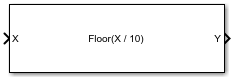# Divide by Constant and Round

Divide input by a constant and round to integer

• Library:
• Fixed-Point Designer

•## Description

The Divide by Constant and Round block outputs the result of dividing the input by a constant and rounds the result to an integer using the specified rounding method.

The Divide by Constant and Round block uses an algorithm that is functionally similar to the Granlund-Montgomery-Warren Method. The division operation is computed via a multiplication by inverse, which generally results in better performance on embedded systems.

## Ports

### Input

expand all

Dividend, specified as a scalar, vector, matrix, or N-D array.

Divide by Constant and Round does not support data types with word length greater than 128.

Data Types: `single` | `double` | `int8` | `int16` | `int32` | `uint8` | `uint16` | `uint32` | `Boolean` | `fixed point`
Complex Number Support: Yes

### Output

expand all

Result of division and round operation, returned as a scalar, vector, matrix, or N-D array.

Data Types: `single` | `double` | `int8` | `int16` | `int32` | `uint8` | `uint16` | `uint32` | `Boolean` | `fixed point`

## Parameters

expand all

Divisor, specified as a positive, real-valued, finite scalar.

#### Programmatic Use

 Block Parameter: `Denominator` Type: character vector Values: MATLAB® expression that evaluates to a positive, real-valued, finite fixed point or numeric value Default: `'10'`

Data Types: `single` | `double` | `int8` | `int16` | `int32` | `int64` | `uint8` | `uint16` | `uint32` | `uint64` | `Boolean` | `fixed point`

Rounding method to use, specified as one of these values:

• `Floor` — Round to nearest integer in the direction of negative infinity.

• `Ceiling` — Round to nearest integer in the direction of positive infinity.

• `Nearest` — Round to the nearest integer. Ties are rounded to the nearest integer in the direction of positive infinity.

• `Zero` — Round to the nearest integer in the direction of zero.

• `Convergent` — Round to the nearest integer. Ties are rounded to the nearest even integer.

#### Programmatic Use

 Block Parameter: `RndMeth` Type: character vector Values: `'Floor'` | `'Ceiling'` | `'Nearest'` | `'Zero'` | `'Convergent'` Default: `'Floor'`

## Extended Capabilities

### Topics

Introduced in R2021a

## Support평가판 신청